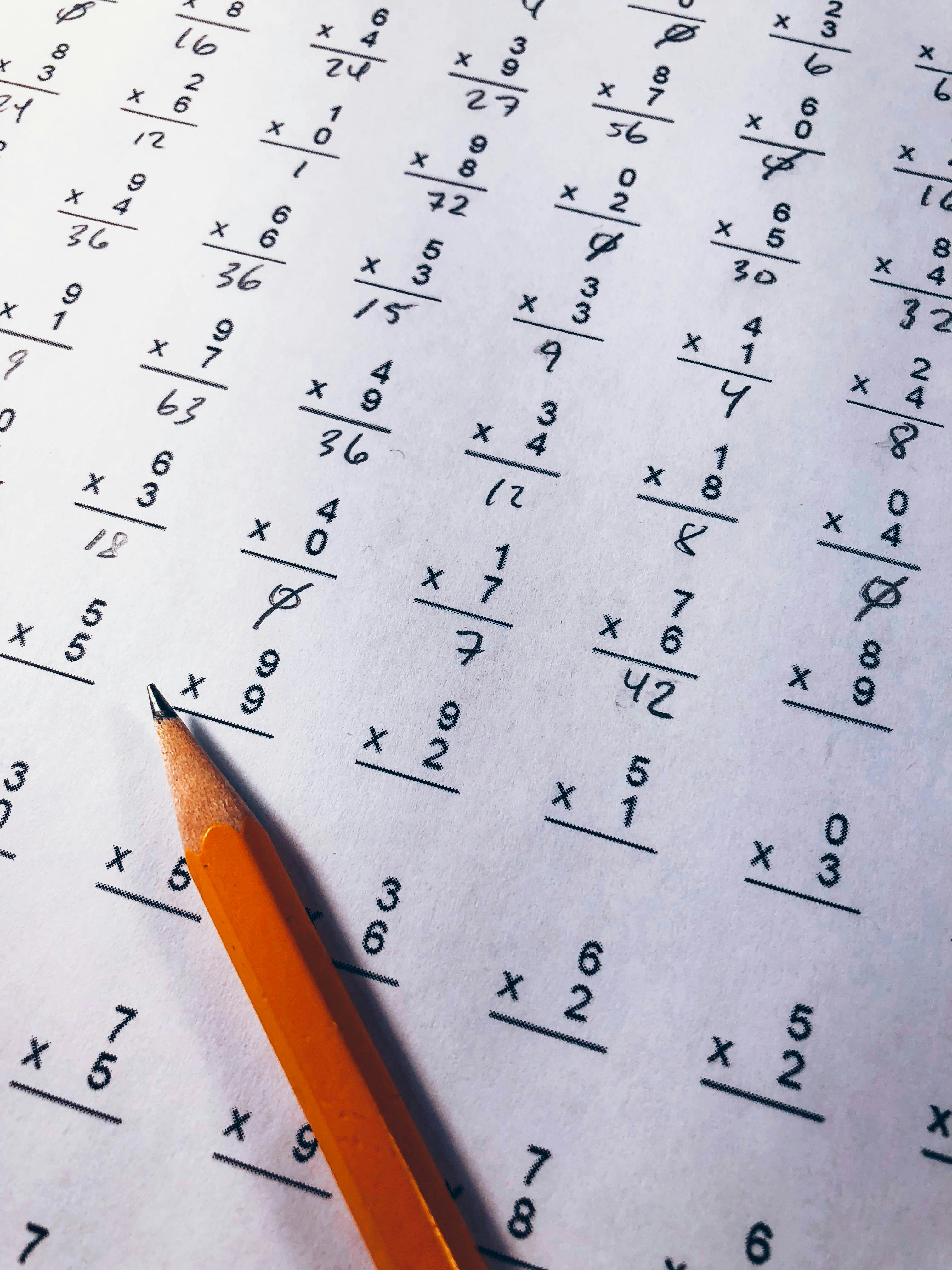Hackerrank - Reduce Function Solution

# Hackerrank - Reduce Function SolutionGiven a list of rational numbers,find their product.

Concept
The reduce() function applies a function of two arguments cumulatively on a list of objects in succession from left to right to reduce it to one value. Say you have a list, say [1,2,3] and you have to find its sum.

>>> reduce(lambda x, y : x + y,[1,2,3])
6

You can also define an initial value. If it is specified, the function will assume initial value as the value given, and then reduce. It is equivalent to adding the initial value at the beginning of the list.

>>> reduce(lambda x, y : x + y, [1,2,3], -3)
3

>>> from fractions import gcd
>>> reduce(gcd, [2,4,8], 3)
1

Input Format

First line contains n, the number of rational numbers.
The  of next  lines contain two integers each, the numerator(  ) and denominator(  ) of the  rational number in the list.

Constraints

Output Format

Print only one line containing the numerator and denominator of the product of the numbers in the list in its simplest form, i.e. numerator and denominator have no common divisor other than .

Sample Input 0

3
1 2
3 4
10 6

Sample Output 0

5 8

Explanation 0

Required product is 1/2 * 3/4 * 10/6 = 5/8

### Solution in Python

from fractions import Fraction
from functools import reduce

def product(fracs):
t = reduce(lambda x,y:x*y, fracs)
return t.numerator, t.denominator

if __name__ == '__main__':
fracs = []
for _ in range(int(input())):
fracs.append(Fraction(*map(int, input().split())))
result = product(fracs)
print(*result)